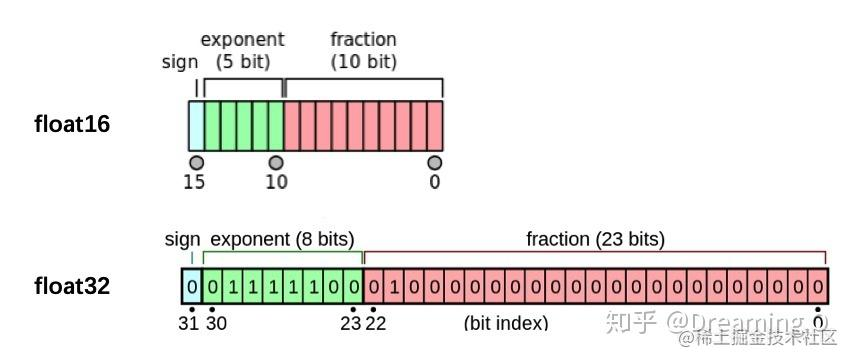# Pytorch学习（六）——

## 6.1 自定义损失函数

PyTorch在torch.nn模块为我们提供了许多常用的损失函数，比如：MSELoss，L1Loss，BCELoss...... 但是随着深度学习的发展，出现了越来越多的非官方提供的Loss，比如DiceLoss，HuberLoss，SobolevLoss...... 这些Loss Function专门针对一些非通用的模型，PyTorch不能将他们全部添加到库中去，因此这些损失函数的实现则需要我们通过自定义损失函数来实现。另外，在科学研究中，我们往往会提出全新的损失函数来提升模型的表现，这时我们既无法使用PyTorch自带的损失函数，也没有相关的博客供参考，此时自己实现损失函数就显得更为重要了。

### 6.1.1 以函数方式定义

python def my_loss(output, target): loss = torch.mean((output - target)**2) return loss

### 6.1.2 以类方式定义

Dice Loss是一种在分割领域常见的损失函数，定义如下：

$$DSC = \frac{2|X∩Y|}{|X|+|Y|}$$ 实现代码如下：

python class DiceLoss(nn.Module): def init(self,weight=None,size_average=True): super(DiceLoss,self).init()

def forward(self,inputs,targets,smooth=1):
inputs = F.sigmoid(inputs)
inputs = inputs.view(-1)
targets = targets.view(-1)
intersection = (inputs * targets).sum()
dice = (2.*intersection + smooth)/(inputs.sum() + targets.sum() + smooth)
return 1 - dice


# 使用方法

criterion = DiceLoss() loss = criterion(input,targets) 

python class DiceBCELoss(nn.Module): def init(self, weight=None, size_average=True): super(DiceBCELoss, self).init()

def forward(self, inputs, targets, smooth=1):
inputs = F.sigmoid(inputs)
inputs = inputs.view(-1)
targets = targets.view(-1)
intersection = (inputs * targets).sum()
dice_loss = 1 - (2.*intersection + smooth)/(inputs.sum() + targets.sum() + smooth)
BCE = F.binary_cross_entropy(inputs, targets, reduction='mean')
Dice_BCE = BCE + dice_loss

return Dice_BCE


class IoULoss(nn.Module): def init(self, weight=None, size_average=True): super(IoULoss, self).init()

def forward(self, inputs, targets, smooth=1):
inputs = F.sigmoid(inputs)
inputs = inputs.view(-1)
targets = targets.view(-1)
intersection = (inputs * targets).sum()
total = (inputs + targets).sum()
union = total - intersection

IoU = (intersection + smooth)/(union + smooth)

return 1 - IoU


ALPHA = 0.8 GAMMA = 2

class FocalLoss(nn.Module): def init(self, weight=None, size_average=True): super(FocalLoss, self).init()

def forward(self, inputs, targets, alpha=ALPHA, gamma=GAMMA, smooth=1):
inputs = F.sigmoid(inputs)
inputs = inputs.view(-1)
targets = targets.view(-1)
BCE = F.binary_cross_entropy(inputs, targets, reduction='mean')
BCE_EXP = torch.exp(-BCE)
focal_loss = alpha * (1-BCE_EXP)**gamma * BCE

return focal_loss


# 更多的可以参考链接1



### 本节参考

【1】https://www.kaggle.com/bigironsphere/loss-function-library-keras-pytorch/notebook
【3】https://blog.csdn.net/dss_dssssd/article/details/84103834
【5】https://blog.csdn.net/qq_27825451/article/details/95165265
【6】https://discuss.pytorch.org/t/should-i-define-my-custom-loss-function-as-a-class/89468

## 6.2 动态调整学习率

### 6.2.1 使用官方scheduler

• 了解官方提供的API

python

# 选择上面提到的一种或多种动态调整学习率的方法

scheduler1 = torch.optim.lr_scheduler.... scheduler2 = torch.optim.lr_scheduler.... ... schedulern = torch.optim.lr_scheduler....

# 进行训练

for epoch in range(100): train(...) validate(...) optimizer.step() # 需要在优化器参数更新之后再动态调整学习率 scheduler1.step() ... schedulern.step() 

### 6.2.2 自定义scheduler

python def adjust_learning_rate(optimizer, epoch): lr = args.lr * (0.1 ** (epoch // 30)) for param_group in optimizer.param_groups: param_group['lr'] = lr

python def adjust_learning_rate(optimizer,...): ... optimizer = torch.optim.SGD(model.parameters(),lr = args.lr,momentum = 0.9) for epoch in range(10): train(...) validate(...) adjust_learning_rate(optimizer,epoch)

【1】PyTorch官方文档

## 6.3 模型微调

### 6.3.1 模型微调的流程

1. 在源数据集(如ImageNet数据集)上预训练一个神经网络模型，即源模型。
2. 创建一个新的神经网络模型，即目标模型。它复制了源模型上除了输出层外的所有模型设计及其参数。我们假设这些模型参数包含了源数据集上学习到的知识，且这些知识同样适用于目标数据集。我们还假设源模型的输出层跟源数据集的标签紧密相关，因此在目标模型中不予采用。
3. 为目标模型添加一个输出⼤小为⽬标数据集类别个数的输出层，并随机初始化该层的模型参数。
4. 在目标数据集上训练目标模型。我们将从头训练输出层，而其余层的参数都是基于源模型的参数微调得到的。### 6.3.2 使用已有模型结构

• 实例化网络

python import torchvision.models as models resnet18 = models.resnet18() # resnet18 = models.resnet18(pretrained=False) 等价于与上面的表达式 alexnet = models.alexnet() vgg16 = models.vgg16() squeezenet = models.squeezenet1_0() densenet = models.densenet161() inception = models.inception_v3() googlenet = models.googlenet() shufflenet = models.shufflenet_v2_x1_0() mobilenet_v2 = models.mobilenet_v2() mobilenet_v3_large = models.mobilenet_v3_large() mobilenet_v3_small = models.mobilenet_v3_small() resnext50_32x4d = models.resnext50_32x4d() wide_resnet50_2 = models.wide_resnet50_2() mnasnet = models.mnasnet1_0() - 传递pretrained参数

python import torchvision.models as models resnet18 = models.resnet18(pretrained=True) alexnet = models.alexnet(pretrained=True) squeezenet = models.squeezenet1_0(pretrained=True) vgg16 = models.vgg16(pretrained=True) densenet = models.densenet161(pretrained=True) inception = models.inception_v3(pretrained=True) googlenet = models.googlenet(pretrained=True) shufflenet = models.shufflenet_v2_x1_0(pretrained=True) mobilenet_v2 = models.mobilenet_v2(pretrained=True) mobilenet_v3_large = models.mobilenet_v3_large(pretrained=True) mobilenet_v3_small = models.mobilenet_v3_small(pretrained=True) resnext50_32x4d = models.resnext50_32x4d(pretrained=True) wide_resnet50_2 = models.wide_resnet50_2(pretrained=True) mnasnet = models.mnasnet1_0(pretrained=True)

1. 通常PyTorch模型的扩展为.pt.pth，程序运行时会首先检查默认路径中是否有已经下载的模型权重，一旦权重被下载，下次加载就不需要下载了。

2. 一般情况下预训练模型的下载会比较慢，我们可以直接通过迅雷或者其他方式去 这里 查看自己的模型里面model_urls，然后手动下载，预训练模型的权重在LinuxMac的默认下载路径是用户根目录下的.cache文件夹。在Windows下就是C:\Users\<username>\.cache\torch\hub\checkpoint。我们可以通过使用 torch.utils.model_zoo.load_url()设置权重的下载地址。

3. 如果觉得麻烦，还可以将自己的权重下载下来放到同文件夹下，然后再将参数加载网络。

python self.model = models.resnet50(pretrained=False) self.model.load_state_dict(torch.load('./model/resnet50-19c8e357.pth'))

1. 如果中途强行停止下载的话，一定要去对应路径下将权重文件删除干净，要不然可能会报错。

### 6.3.3 训练特定层

python def set_parameter_requires_grad(model, feature_extracting): if feature_extracting: for param in model.parameters(): param.requires_grad = False

python import torchvision.models as models

# 冻结参数的梯度

feature_extract = True model = models.resnet18(pretrained=True) set_parameter_requires_grad(model, feature_extract)

# 修改模型

num_ftrs = model.fc.in_features model.fc = nn.Linear(in_features=512, out_features=4, bias=True) 

【1】参数更新
【2】给不同层分配不同的学习率

## 6.4 半精度训练• 如何在PyTorch中设置半精度训练
• 使用半精度训练的注意事项

### 6.4.1 半精度训练的设置

• import autocast

python from torch.cuda.amp import autocast

• 模型设置

python @autocast() def forward(self, x): ... return x

• 训练过程

python for x in train_loader: x = x.cuda() with autocast(): output = model(x) ...`

「其他文章」